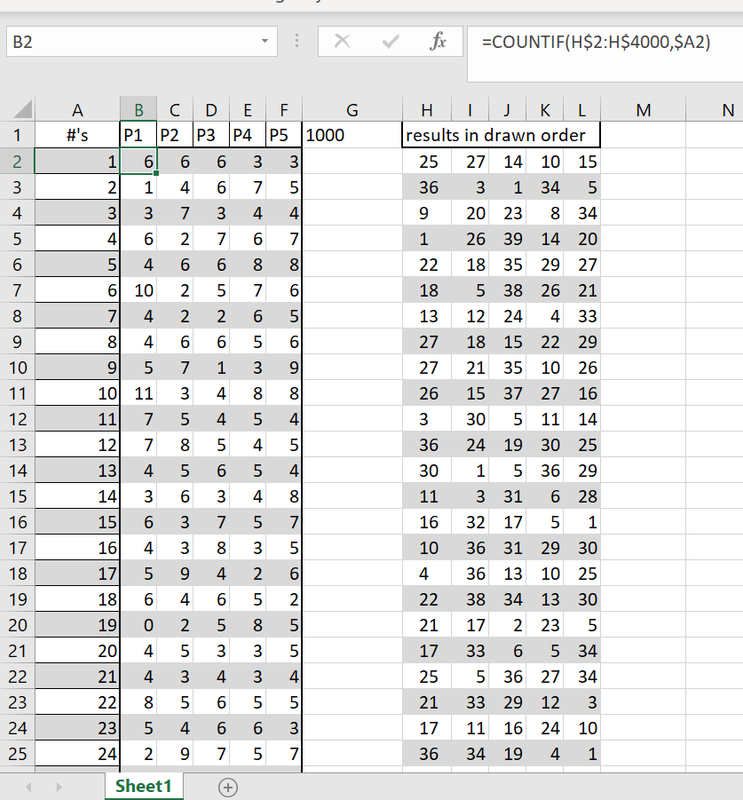# Lottery Grid

#### Winalot2007

##### Member
Looking for help creating a grid that will count how many numbers by position appear in a lottery game matrix
For example,
Lottery Game 5/39
Cell A1 = #'s
Cell range A2 through A40 = lottery numbers 1 through 39
Cell B1 = P1 Position 1
Cell C1 = P2 Position 2
Cell D1 = P3 Position 3
Cell E1 = P4 Position 4
Cell F1 = P5 Position 5

Starting in cell B2 - formula that calculates how many times the #1 appears in all the sets for just Position 1
Continue down for the remaining numbers in cell range B3:B40
Thanks

#### Frank

##### MemberYou dont say where your data set of results are. This will affect the formula you create.
Assuming that your data set is in columns H to L and in drawn order ( there would be no point in counting otherwise) , starting at row 2 downwards,and assuming your data has less than 4000 rows:-

in cell B2 you need the formula =COUNTIF(H\$2:H\$4000,\$A2).

In the formula H\$2 anchors the top left cornerof the range being counted to that cell, so that copying the formula along and down will not move that reference cell. \$A2 does the same thing, anchoring what is being counted to column 1 even if you copy the formula across the row. Note that you need to modify H\$4000 if you have more rows of data than 4000 of if you have some other data below and within counting range, or if the top left of your data is not in column H.

The formula looks down column B and counts ocurrences of the item in A2 which is a 1. Copy that formula along the row to column F. This row now counts ocurrences of number 1 in each position. Copy that row of 5 formulas down to row 40, and the grid now counts all 39 numbers in each of the positions. If you want a checksum, the sum of all these counts... in cell G1, formula := =SUM(B2:F40) should equal (number of draws x 5). My test data has 200 draws, so this check sum is correct.
I hope this is what you were looking for.